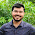Wednesday, October 14, 2015

Velocity of Projectile and Problems on Projectile Motion

A projectile is a body that is having a two-dimensional motion. A body thrown, making an angle with the horizontal other than 90° can have a two-dimensional motion. We shall project the body with a initial velocity for this to happen. This initial velocity can be resolved into horizontal and vertical components. There is no influence of acceleration due to gravity along the horizontal direction and hence velocity component along the horizontal direction always remains constant. As the gravity acts along the Y direction, the velocity component along the Y direction keeps changing with respect to time. Here we are going to find out the effect to velocity of the projectile after a specified time.

Let us consider a body is projected with the initial velocity making an angle with the horizontal. We can calculate the horizontal and vertical components of velocities of the body using the equation of motion. Being these two are perpendicular components to each other, using the parallelogram law that starts with can find the effective velocity of the projectile as shown below. It can be noticed that at the maximum height vertical component of the velocity zero and hence the velocity of the projectile is equal to the horizontal component of velocity itself. And hence projectile will have least possible allows state the maximum height but that is not equal to 0. We can also calculate the change in the moment of the projectile between the two points of its initial and final journey.

Let us calculate the time after which initial and final the last is of the projectile are perpendicular to each other. We can write the velocity of the projectile in vector format as shown below. Both initial and final velocities of the projectile were returned the vector formats. We know that if two vectors are perpendicular to each other their scalar product has to be zero. Hence we can find the scalar product of the initial and final at the arts of the projectile and we can equate to 0. By doing this we can identify the time after which initial and final velocity of the projectile are perpendicular to each other as shown below.

Using the similar sort of concept we can also calculate the velocity of the projectile at half of its maximum height. Even in this case the horizontal component of velocity remains constant. We can find out the vertical component of the velocity at the half of the maximum height using the third equation of motion of kinematics as shown below. Anyway these two components are always perpendicular to each other and by using the parallelogram law sectors we can find the resultant vector as shown below.

Problem on velocity of projectile

A body is projected with a initial velocity and by making an angle with the horizontal. The body makes 30° angle with the horizontal after two seconds and then after one second it reaches the maximum height. What is the angle of projection and what is the speed of the projectile?

Solution

We know that horizontal and vertical components of elasticity different and we can find the angle between them using a trigonometrical  equation. By equating this value with the 30° with can get one relation. When the body reaches the maximum height the angle made by the body with the horizontal is zero and again by equating with this value with can get one more a question. By solving these two equations we can get the velocity of the projectile and angle of the projectile as shown below.

Problem on projectile motion

A football is kicked with a initial velocity of 19.6 m/s with an angle of projection of 45 degree by one player. On the goal line 67.4 m away from this player and from the direction of the click, another player start running to meet the ball at the same instant. What must be his speed to catch the ball before it lands in the goal post ?

Solution

We know that as the ball is projected with a 45° angle it’s angle of projection helps the body to reach its maximum range and we can calculate the maximum range using its formula as the 39.2 m. And we can also calculate the time taken by the body to reach the ground using the time of fight formula as shown below. If the other player has to catch the ball he has to reach the ball before it strikes the ground in the same time that is equal to time of flight. As he is running on the ground with a uniform velocity we can use a simple formula that the distance that it has to cover is equal to product of velocity and time. Hence we can solve the problem as shown below.

Expression of range and maximum height in terms of constants of the parabolic equation

we can express range and maximum height in terms of constants of the parabola as shown below.

Related Posts

1 comment:

1.two particles projected in opposite directions horizontally from a given point in space where gravity g is uniform. If u1 and u2 are their initial speeds then what is the time after which their velocities are mutually perpendicular?